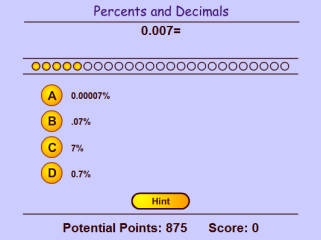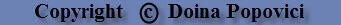# MATH-PLAY.COM## DECIMALS AND PERCENTS GAME

In this decimals and percents game, you have to change decimals to percents and vice-versa. To get a lot of points, you have to answer math questions quickly and correctly. Hints are available, but you will lose 300 points for each hint.

This game is suitable for fifth grade and middle school students.

Note: This activity works on computers and laptops, but not iPads or other tablets.This game is aligned to the following Common Core Math Standards: CCSS.Math.Content.5.NBT.A.2 Explain patterns in the number of zeros of the product when multiplying a number by powers of 10, and explain patterns in the placement of the decimal point when a decimal is multiplied or divided by a power of 10. Use whole-number exponents to denote powers of 10. CCSS.Math.Content.5.NBT.A.3 Read, write, and compare decimals to thousandths.

Visit this link to play other middle school math games.

Return from this Decimals and Percents activity to the Math Play homepage.